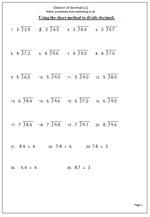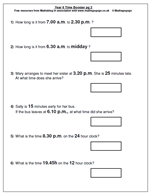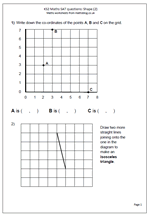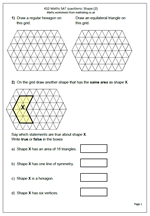## Resource of the Week: short division of decimalsThis week I would like to look at a page of practice on using the short method of division of decimals. The numbers being divided are just units and tenths which helps with getting the method correct.

There are arguments for and against putting the decimal point in before you start, or leaving it until you have reached that point in the question. it does not matter as long as it is inserted correctly.

One of the best ways to be fluent with this method is to talk it through out loud. If we look at question 2 which is 4.5 divided by 3, the verbal stages are:

a. How many 3s in 4?

b. 1 times 3 is 3 so there is 1 with a remainder of 1.

c. Place the 1 on the answer line, immediately above the 3.

d. Place the decimal point just above the answer line so it can be clearly seen.

e. The remainder 1 is placed just in front of the 5 (usually written smaller).

f. How many 3s in 15?

g. 3 x 5 is 15 so the answer is 5.

h. Place the 5 on the answer line, immediately above the 5 (tenths).

Division of decimals (1)

## Year 6 SAT questionsWith SATs just a couple of weeks away now is the  time of year that schools intensify their year 6 revision.. The types of question that come up are fairly predictable and follow a similar layout each year. It is well worthwhile, therefore, to let your children have a practice at the style of questions they are likely to come across on the test.

We have a good selection of SAT questions which can be printed free of charge, including pages on:

writing numbers

making mathematical statements true

completing number sentences

number problems

time problems

symmetry

Why not have a look at our Year 6 ‘Booster’ pages for SATs?

## KS2 SAT revision: Shape (3)Another in our set of KS2 SAT revision worksheets on shape. This page looks at the understanding of co-ordinates and shape. The first question is straightforward and has three points on the grid to write the co-ordinates for. Now many children come across an easy question like this and instantly forget which number should come first. They need a simple memory nudge to remind them such as, ‘along the corridor and up the stairs’, although there are many others as well. In this case the brackets and comma are provided, but this might not always be the case and children are expected to use these correctly.

The second question is testing whether children understand what an isosceles triangle is. The grid is provided, but this can sometimes cause confusion. Once again, go for the most obvious way of doing this.

Whilst these questions will not teach the concepts they do act as a quick test as to whether or not more time needs to be spent on shape.

This, an other similar pages can be found in our Key Stage 2 Maths SAT Questions category.

KS 2 Maths SAT questions_Shape (3)

## KS2 SATs revision: Shape (2)With KS2 SATs coming up soon it is about time I added a few more worksheets to help. This one shows a typical couple of questions on shape. They are fairly straightforward and would be an easy way to collect marks towards Level 4.

The first question is really testing whether the terms regular hexagon and equilateral triangle are understood. Each of these will have sides of equal length.

The second question covers several concepts, including measuring area, lines of symmetry and the terms hexagon and vertices.

From these questions it is easy to see why there are sometimes discrepancies in marking. There are many answers to drawing a shape with the same area as X. Markers of the test papers would have to spend time counting the triangles to make sure, something many would be reluctant to do as it takes time and they will want to get them marked as quickly as possible, so a complex shape may be dismissed. So, keep the answers simple and obvious.

KS 2 Maths SAT questions_Shape (2)

## Booster maths worksheet 11

The writers of the SATs papers love little puzzles such as the square shown on this worksheet.Those lovely people who write the SATs really like little mathematical puzzles. One such puzzle is to fill in missing numbers in a square or block. There will always be a logical way to find the answers, usually by completing what is possible will lead to the creation of the next possible step until the whole square can be completed.

They also like questions with multiple steps and many possible answers: question 2 is one such. The approach here is to add the two given numbers (300 + 600 = 900) and then subtract the 900 from the total of 1200. The two numbers have to add up to 300 and could be any combination. Taken step by step this is not difficult but as I have stated before, many children find it very difficult indeed to work through to an answer if it requires more than one step.

Booster maths worksheet 11